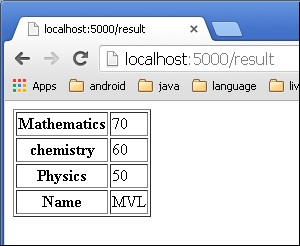# Flask Form Data to Template

Estimated reading: 2 minutes 580 views

We have already seen that the http method can be specified in URL rule. The Form data received by the triggered function can collect it in the form of a dictionary object and forward it to a template to render it on a corresponding web page.

In the following example, ‘/’ URL renders a web page (student.html) which has a form. The data filled in it is posted to the ‘/result’ URL which triggers the result() function.

The results() function collects form data present in request.form in a dictionary object and sends it for rendering to result.html.

The template dynamically renders an HTML table of form data.

Given below is the Python code of application −

```				```

@app.route('/')
def student():
return render_template('student.html')

@app.route('/result',methods = ['POST', 'GET'])
def result():
if request.method == 'POST':
result = request.form
return render_template("result.html",result = result)

if __name__ == '__main__':
app.run(debug = True)
```
```

Given below is the HTML script of student.html.

```				```
<html>
<body>
<form action = "http://localhost:5000/result" method = "POST">
<p>Name <input type = "text" name = "Name" /></p>
<p>Physics <input type = "text" name = "Physics" /></p>
<p>Chemistry <input type = "text" name = "chemistry" /></p>
<p>Maths <input type ="text" name = "Mathematics" /></p>
<p><input type = "submit" value = "submit" /></p>
</form>
</body>
</html>
```
```

Code of template (result.html) is given below −

```				```
<!doctype html>
<html>
<body>
<table border = 1>
{% for key, value in result.items() %}
<tr>
<th> {{ key }} </th>
<td> {{ value }} </td>
</tr>
{% endfor %}
</table>
</body>
</html>
```
```

Run the Python script and enter the URL http://localhost:5000/ in the browser.When the Submit button is clicked, form data is rendered on result.html in the form of HTML table.